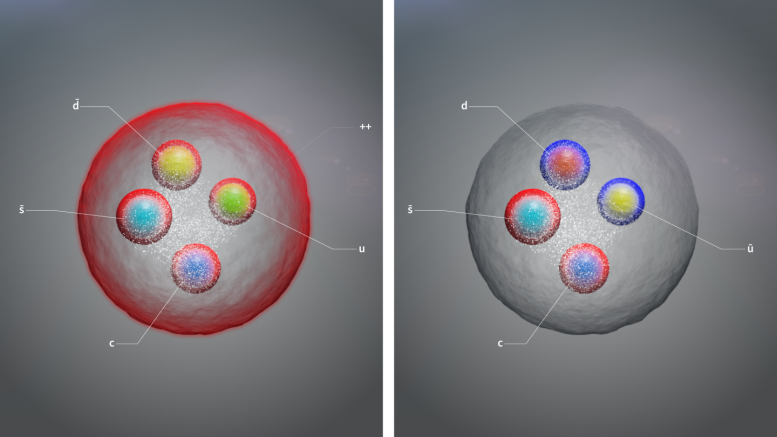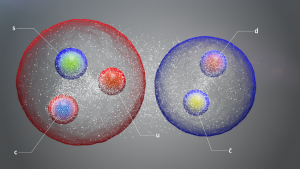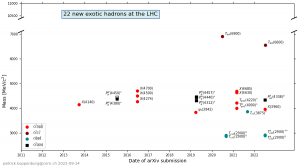# Exotic Observations: LHCb Discovers Pentaquark and Tetraquark PairThe Large Hadron Collider beauty (LHCb) experiment reports observation of an exotic tetraquark hadron pair (illustrated). The pair consists of a $$c\overline{s}u\overline{d}$$ (left) and a $$c\overline{s}\overline{u}d$$ (right), with the former retaining a double electrical charge (++) and the latter, its electrically neutral counterpart. The two new particles are also the first tetraquark pair ever observed | Source: CERN

On July 05, 2022, collaborators from Tsinghua University (CN), Università degli Studi (IT), and INFN Milano (IT) presented findings at an LHC seminar with new observations of exotic particles, on behalf of the Large Hadron Collider beauty (LHCb) collaboration. The report, Particle Zoo 2.0: New Tetra- and Pentaquarks at LHCb, describes the new pentaquark with strangeness (doubly charged/neutral tetraquarks) main decay channels through multibody decays of $$B^- (B^{+/0})$$ mesons with a $$J/\psi \Lambda \pi (DD_s^+ \overline{\rho})$$ final state. The results were reported with $$15$$ standard deviations ($$\sigma$$) for the pentaquark, $$6.5 \sigma$$ for the doubly charged tetraquark, and $$8\sigma$$ for the neutral tetraquark (for reference, $$5\sigma$$ is the standardized minimum for a particle observation declaration in particle physics). The experimental data posits valuable insight into the theoretical structure of such multiquark hadrons, especially with regard to whether they group as $$SU(3)$$ multiplets (tight quark clusterings) or as pseudo-molecular states with distinct smaller interacting clusters.An illustration of the proposed pseudo-molecular formation for the uscc̄d pentaquark discovered at LHCb. The model proposes a distinct departure from the standard multiplet-cluster structure used to define triquark baryons such as nucleons. | Source: CERN

The six quarks of the Standard Model (SM)—up ($$u$$), down ($$d$$), charm ($$c$$), strange ($$s$$), top ($$t$$), and bottom ($$b$$)—along with their respective antiquarks, are the fundamental building blocks of hadronic matter. Groupings of these quarks, held together by the strong nuclear force through excitations in the gluon field (or gluon particles/flux-tubes in a perturbative approach), are prone to an excited series of radiation and pair production, through the hadronization governed by quantum chromodynamics (QCD). The most well-known hadrons, protons and neutrons, have triquark compositions (in a subclass known as baryons), with the former showing its positive charge from two up quarks ($$+2/3e$$) and one down ($$−1/3e$$), while the latter displays neutral electromagnetic charge as a result of its two down, one up composition. These hadrons, when unified with electrons (generation $$1$$, charged leptons) in an atomic structure, form the fundamental building blocks of luminiferous (standard) matter as most commonly observed by humans.

While the electromagnetic charge-composition of hadrons is defined solely by the clustered quarks involved, a hadron’s strong charge, dubbed “color charge,” relies on both quarks and gluons. Color charge comes in 3 states, along with 3 corresponding anti-states: red, green, and blue. The basic way to determine a composite particle’s color is to simply sum the charges assuming red $$+$$ green $$+$$ blue $$= 0$$ and red $$+$$ anti-red $$= 0$$ (neutral).

The behavior of the colors is defined in QCD by a special unitary symmetry group of degree $$3$$, $$SU(3)$$, where gluons are the basis states of the Lie algebra. That is to say, the strong force state of a particle is given by a vector where the corresponding vector space has elements of $$SU(3)$$ behaving as unitary operators. For reference, a unitary group of degree $$n$$, $$U(n)$$, has elements of $$n\times n$$ unitary matrices, while preserving the standard inner product of the complex $$n$$-tuples. A special unitary group of degree $$n$$, $$SU(n)$$, is a subgroup whose element matrices have determinant 1. Because the elements of $$SU(3)$$ are $$3\times 3$$ matrices, they can transform $$3$$-dimensional particle state vectors by matrix multiplication. Under such transformations, it turns out quarks’ color really is just a pneumonic for the column vectors such that red is $$[1, 0, 0]$$, green is $$[0, 1, 0]$$, and blue is $$[0, 0, 1]$$, and are acted on by the $$SU(3)$$ elements via left multiplication. Similarly, the anti-colors can be represented by corresponding row vectors and interact with elements of $$SU(3)$$ through right multiplication. The mathematical representation of a gluon’s charge is intuitively given by a $$3\times 3$$ matrix with a single non-zero entry, thus giving it a color and an anti-color (as an $$n^{\text{th}}$$ column entry is simultaneously an $$m^{\text{th}}$$ row entry).A table depicting the color combinations of gluons as elements of $$SU(3)$$. Note that these elements are generated via the Gell-Mann traceless Hermitian matrices, of which there exists 8. Taking linear combinations of the generators cannot yield a 1 in the top-right entry, disallowing a red, anti-red gluon.| Typesetting: Dheeran Wiggins | Source: UCR

In order for a cluster of these particles to form a somewhat stable hadron, such that the individual quarks or gluons do not immediately hadronize into jets, the net color of the cluster must be neutral. Consequently, there are only certain combinations of quarks and gluons that can form together, though the limitations of number of quarks depends mostly on probability of occurrence. While the most commonly observed hadrons are in diquark pairs or triquark clusters, mesons (even-degree hadrons) of degree $$>2$$ and baryons (odd) of degree $$>3$$ have been observed in experiments; these are called exotic multiquark hadrons.

Experimental Findings & Future Prospects

The physicists at Tsinghua and INFN reported resonances for a tetraquark pair at $$M \approx 2.9\text{ GeV}$$ and a pentaquark at $$M \approx 4338.2 ± 0.7 ± 0.4 \text{ MeV}$$ in data sets collected from runs at the LHCb. These resonant peaks on event histograms suggest a statistical signal for the mass of the particle being searched for at an experiment, and when the standard deviations are taken beyond 5σ, they are reported by the experiment as a confirmed particle mass. The collaboration also reports decay widths of $$\Gamma \approx 136 \text{ MeV}$$ and $$\Gamma \approx 7.0 \pm 1.2 \pm 1.3 \text{MeV}$$ respectively. The decay width of a particle is equivalent to the reciprocal of its lifetime ($$\tau$$) such that $$\Gamma \propto 1/\tau$$. This value represents a measure of the specific decay mechanism occurring in a given time relative to the parent (decayed from) particle’s reference frame.The 22 exotic hadrons observed in experiments at the LHC at given mass levels, distributed by year of publication preprint submitted to the arXiv. | Source: Patrick Koppenburg, LHCb

After the observation of these three new multiquark hadrons, the collection of exotic experimental findings at LHCb only continues to grow, currently sitting at 22 in the last 10 years. Other potential exotic candidates from the search—such as the $$\chi (3960)$$ in a $$D_s^+ D_s^-$$ decay—are relatively close to the discovery threshold of $$5\sigma$$. Such “threshold” exotics remain a primary target for future analyses, usually requiring higher luminosity runs (suggesting potential HL-LHC upgrades) or simply increased event-frequency/provenance statistics. Additionally, further observations of exotic hadrons could provide insight into the fundamental makeup of the quarks within the overall cluster, as preferred decay mechanisms could imply either $$SU(3)$$ multiplets (like nucleons) or pseudo-molecular formations.